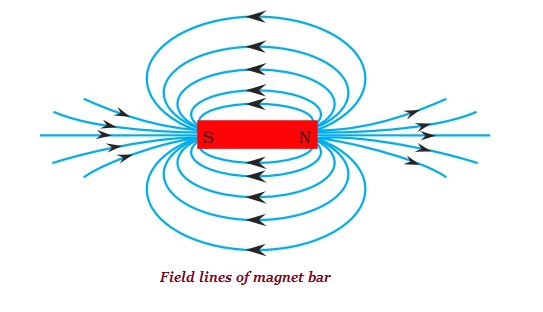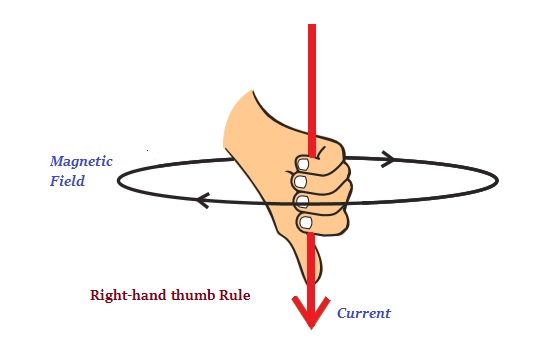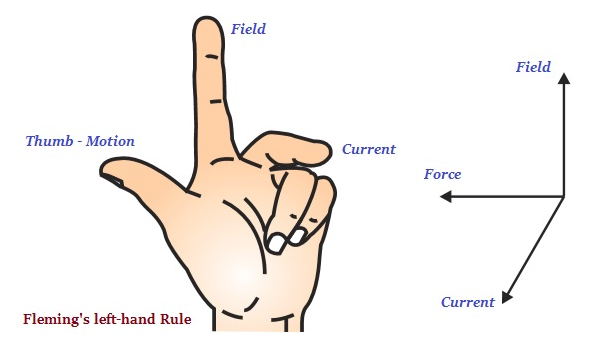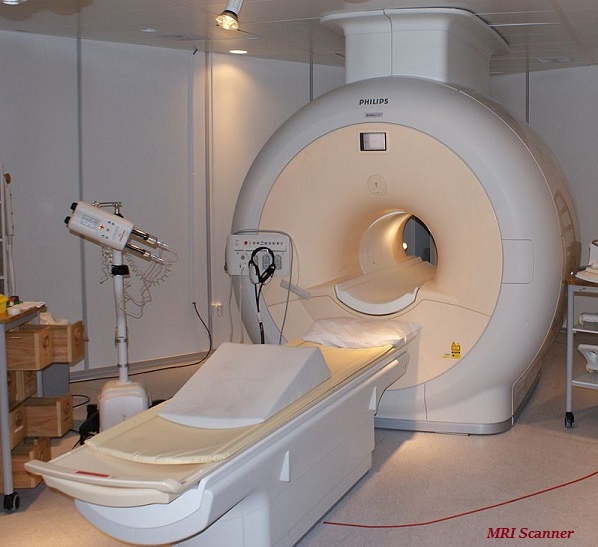# Physics - Magnetic Effects of Electric Current

## Introduction

• The electricity and magnetism are linked to each other and it is proved when the electric current passes through the copper wire, it produces a magnetic effect.

• The electromagnetic effects first time noticed by Hans Christian Oersted.

## Magnetic Field

• Magnetic field is a quantity, which has both magnitude and direction.

• The direction of a magnetic field is usually taken to be the direction in which, a north pole of the compass needle moves inside it.• It is the convention that the field lines emerge from north pole and merge at the south pole (see the image given above).

• No two field-lines of a magnet bar are found to cross each other. If it happens, then it means that at the point of intersection, the compass needle would point towards two directions, which is simply not possible.

• The magnitude of the magnetic field (produced by an electric current) at a given point increases with the increase of current through the wire.

## Right-Hand Thumb Rule

• Also known as Maxwell’s corkscrew rule, right-hand thumb rule illustrates direction of the magnetic field associated with a current-carrying conductor (see the image given below).• Right-hand thumb rule states that “Imagine that you are holding a current-carrying straight conductor in your right hand such that the thumb points towards the direction of current. Then your fingers will wrap around the conductor in the direction of the field lines of the magnetic field.”

## Fleming’s Left-Hand Rule

• Fleming’s left-hand rule states that “Stretch the thumb, forefinger and middle finger of your left hand such that they are mutually perpendicular (as shown in the image given below). If the first finger points in the direction of magnetic field and the second finger in the direction of current, then the thumb will point in the direction of motion or the force acting on the conductor.”• Human body also produces magnetic field; however, it is very weak and about one-billionth of the earth’s magnetic field.

• Heart and brain are the two main organs in the human body where the magnetic field has been produced.

• The magnetic field inside the human body forms the basis of getting the images of different parts of the body.

• The technique used to get the image of body part is known as the Magnetic Resonance Imaging (MRI).